# Sinusoid

(diff) ← Older revision | Latest revision (diff) | Newer revision → (diff)

The graph of the function(see Fig.). The sinusoid is a continuous curve with period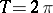. It intersects the-axis at the points. These are also points of inflection, meeting the-axis at an angle of. The extrema are at the points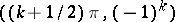.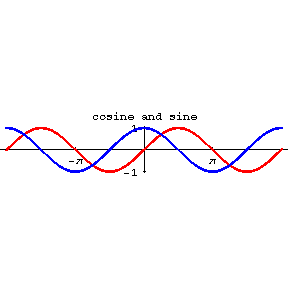Figure: s085640a

The graph of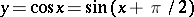is the cosinusoid, obtained by shifting the sinusoid a distance ofto the left. The cosinusoid intersects the-axis at the points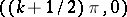, and its extrema are at the points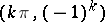.

Many oscillatory processes can be described by a periodic function of the form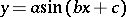, where,andare constants and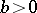. The graph of this function (called a general sinusoid) is obtained from the graph of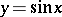(the ordinary sinusoid) as follows: expand in the direction of the-axis by a factor, contract in the-direction by a factor, translate to the left over a distance, and when: reflect in the-axis. Its period is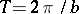and it meets the-axis at the points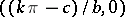. Its extrema are at the points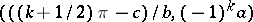.

In this article,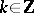.